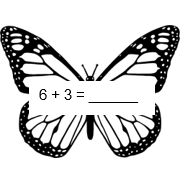# Lesson Plan: Insects (Math - Grade 2)Subject:  Math

Lesson Objective: To practice math facts using addition and subtraction

Common Core Standard:  CCSS.MATH.CONTENT.2.OA.B.2- Fluently add and subtract within 20 using mental strategies.

Materials:

Starter:

Say:

• What is the difference between addition and subtraction? (Allow the students to answer.)

Main:

Say:

• Addition and subtraction are strategies that we use to find out how many total items there are or how many items have been taken away.
• Addition is used when you are putting two numbers together.  You might have two plates of cookies and you want to know how many total cookies you have.  You would use addition to figure that out.
• Subtraction is used when you are taking a number away from another number or trying to figure out the difference.  You might see two plates of cookies, but one plate has more cookies on it.  You could use subtraction to figure out how many more cookies one plate has than the other.
• There are many addition and subtraction facts.  Facts are addition or subtraction problems that you can memorize.  Facts usually add or subtract smaller numbers.
• It is so important to know addition and subtraction facts.  The faster you can answer facts, the easier math will be.
• For example, 2 + 2 is an addition fact.  You can memorize this and easily know that the answer is 4.
• Another example is 10 – 5.  This is a subtraction fact.  You can memorize this and easily know that the answer is 5.
• Now, you are going to do a worksheet with addition and subtraction facts.  Do your best to answer using your memory and not your fingers or number line.  When you are done, we will go over all of the answers.
• Does anyone have any questions?

Feedback:

Say:

• Who would like to share your answers?  (Allow the students to share.)

Related lessons:

Emergency Sub Plan: Insects - Science

Emergency Sub Plan: Insects - Reading

Emergency Sub Plan: Insects - Math

Check out our complete Emergency Sub Plans Library!

Written by Kimberly Greacen, Education World® Contributing Writer

Kimberly is an educator with extensive experience in curriculum writing and developing instructional materials to align with Common Core State Standards and Bloom's Taxonomy.